# Avinash Sharma V - «Understanding activation functions in neural networks» (2017-03-31)

## Activation functions

So what does an artificial neuron do?
Simply put, it calculates a “weighted sum” of its input, adds a bias and then decides whether it should be “fired” or not.

So consider a neuron.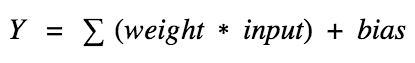Now, the value of Y can be anything ranging from -inf to +inf. The neuron really doesn’t know the bounds of the value.
So how do we decide whether the neuron should fire or not?
We decided to add “activation functions” for this purpose.
To check the Y value produced by a neuron and decide whether outside connections should consider this neuron as “fired” or not. Or rather let’s say — “activated” or not.

## Step function

The first thing that comes to our minds is how about a threshold based activation function?

• If the value of Y is above a certain value, declare it activated.
• If it’s less than the threshold, then say it’s not.

Activation function A = “activated” if Y > threshold else not.
Alternatively, A = 1 if y> threshold, 0 otherwise

Well, what we just did is a “step function”, see the below figure: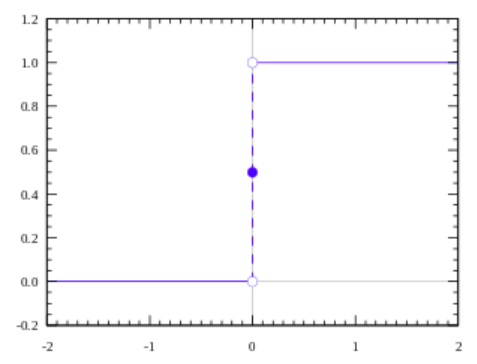Its output is 1 ( activated) when value > 0 (threshold) and outputs a 0 ( not activated) otherwise.

However, there are certain drawbacks with this.
Suppose you are creating a binary classifier.
Something which should say a “yes” or “no” ( activate or not activate ).
A Step function could do that for you!
That’s exactly what it does, say a 1 or 0.
Now, think about the use case where you would want multiple such neurons to be connected to bring in more classes. Class1, class2, class3 etc.
What will happen if more than 1 neuron is “activated”?
All neurons will output a 1 ( from step function).
Now what would you decide? Which class is it? Hmm hard, complicated.

You would want the network to activate only 1 neuron and others should be 0 (only then would you be able to say it classified properly/identified the class ).
Ah! This is harder to train and converge this way.
It would have been better if the activation was not binary and it instead would say “50% activated” or “20% activated” and so on.
And then if more than 1 neuron activates, you could find which neuron has the “highest activation” and so on (better than max, a softmax, but let’s leave that for now).

In this case as well, if more than 1 neuron says “100% activated”, the problem still persists.
I know! But…since there are intermediate activation values for the output, learning can be smoother and easier (less wiggly) and chances of more than 1 neuron being 100% activated is lesser when compared to step function while training (also depending on what you are training and the data).

Ok, so we want something to give us intermediate (analog) activation values rather than saying “activated” or not (binary).

The first thing that comes to our minds would be Linear function.

## Linear function

``````A = cx
``````

A straight line function where activation is proportional to input (which is the weighted sum from neuron).

This way, it gives a range of activations, so it is not binary activation.
We can definitely connect a few neurons together and if more than 1 fires, we could take the max (or softmax) and decide based on that.
So that is ok too.
Then what is the problem with this?

If you are familiar with gradient descent for training, you would notice that for this function, derivative is a constant.

`A = cx`, derivative with respect to `x` is `c`.
That means, the gradient has no relationship with X.
It is a constant gradient and the descent is going to be on constant gradient.
If there is an error in prediction, the changes made by back propagation is constant and not depending on the change in input delta(x).

There is another problem too.
Each layer is activated by a linear function.
That activation in turn goes into the next level as input and the second layer calculates weighted sum on that input and it in turn, fires based on another linear activation function.

No matter how many layers we have, if all are linear in nature, the final activation function of last layer is nothing but just a linear function of the input of first layer!

That means these two layers (or N layers) can be replaced by a single layer.
Ah! We just lost the ability of stacking layers this way.
No matter how we stack, the whole network is still equivalent to a single layer with linear activation ( a combination of linear functions in a linear manner is still another linear function).

## Sigmoid Function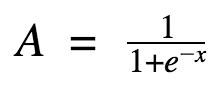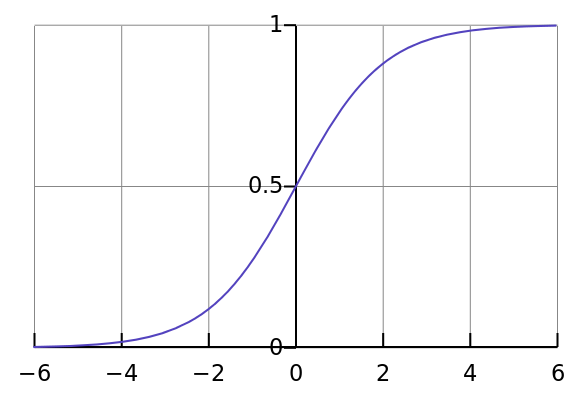Well, this looks smooth and “step function like”.
What are the benefits of this?
First things first, it is nonlinear in nature. Combinations of this function are also nonlinear! Great. Now we can stack layers.
What about non binary activations? Yes, that too!. It will give an analog activation unlike step function. It has a smooth gradient too.

And if you notice, between X values -2 to 2, Y values are very steep.
Which means, any small changes in the values of X in that region will cause values of Y to change significantly.
Ah, that means this function has a tendency to bring the Y values to either end of the curve.

Looks like it’s good for a classifier considering its property? Yes ! It indeed is.
It tends to bring the activations to either side of the curve (above x = 2 and below x = -2 for example). Making clear distinctions on prediction.

Another advantage of this activation function is, unlike linear function, the output of the activation function is always going to be in range (0,1) compared to (-inf, inf) of linear function.
So we have our activations bound in a range. Nice, it won’t blow up the activations then.

This is great. Sigmoid functions are one of the most widely used activation functions today. Then what are the problems with this?

If you notice, towards either end of the sigmoid function, the Y values tend to respond very less to changes in X. What does that mean?
The gradient at that region is going to be small. It gives rise to a problem of “vanishing gradients”. Hmm. So what happens when the activations reach near the “near-horizontal” part of the curve on either sides?

Gradient is small or has vanished (cannot make significant change because of the extremely small value). The network refuses to learn further or is drastically slow (depending on use case and until gradient /computation gets hit by floating point value limits). There are ways to work around this problem and sigmoid is still very popular in classification problems.

## Tanh Function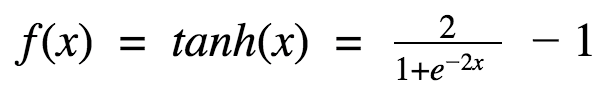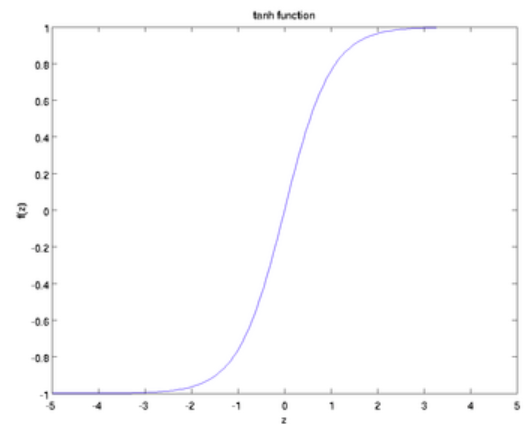Hm. This looks very similar to sigmoid. In fact, it is a scaled sigmoid function!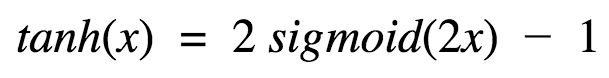Ok, now this has characteristics similar to sigmoid that we discussed above.

• It is nonlinear in nature, so great we can stack layers!
• It is bound to range (-1, 1) so no worries of activations blowing up.

One point to mention is that the gradient is stronger for tanh than sigmoid (derivatives are steeper).
Deciding between the sigmoid or tanh will depend on your requirement of gradient strength.
Like sigmoid, tanh also has the vanishing gradient problem.

Tanh is also a very popular and widely used activation function.

## ReLU

``````A(x) = max(0,x)
``````

The ReLu function is as shown above. It gives an output x if x is positive and 0 otherwise.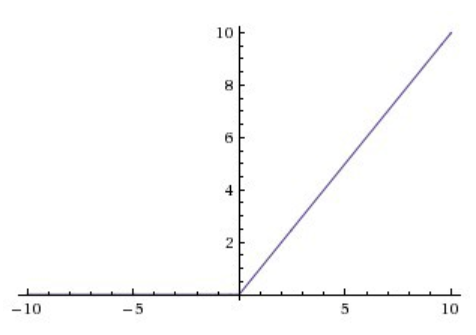At first look this would look like having the same problems of linear function, as it is linear in positive axis.
First of all, ReLu is nonlinear in nature.
And combinations of ReLu are also non linear!
In fact it is a good approximator. Any function can be approximated with combinations of ReLu.
Great, so this means we can stack layers.

It is not bound though. The range of ReLu is [0, inf).
This means it can blow up the activation.

Another point that I would like to discuss here is the sparsity of the activation.
Imagine a big neural network with a lot of neurons.
Using a sigmoid or tanh will cause almost all neurons to fire in an analog way (remember?).
That means almost all activations will be processed to describe the output of a network.
In other words the activation is dense.
This is costly.
We would ideally want a few neurons in the network to not activate and thereby making the activations sparse and efficient.
ReLu give us this benefit.
Imagine a network with random initialized weights (or normalised) and almost 50% of the network yields 0 activation because of the characteristic of ReLu (output 0 for negative values of x).
This means a fewer neurons are firing (sparse activation) and the network is lighter.
Woah, nice! ReLu seems to be awesome! Yes it is, but nothing is flawless. Not even ReLu.

Because of the horizontal line in ReLu( for negative X), the gradient can go towards 0.
For activations in that region of ReLu, gradient will be 0 because of which the weights will not get adjusted during descent.
That means, those neurons which go into that state will stop responding to variations in error/ input (simply because gradient is 0, nothing changes).
This is called dying ReLu problem.
This problem can cause several neurons to just die and not respond making a substantial part of the network passive.

There are variations in ReLu to mitigate this issue by simply making the horizontal line into non-horizontal component.
For example y = 0.01x for x<0 will make it a slightly inclined line rather than horizontal line. This is leaky ReLu.

There are other variations too.
The main idea is to let the gradient be non zero and recover during training eventually.

ReLU is less computationally expensive than tanh and sigmoid because it involves simpler mathematical operations.
That is a good point to consider when we are designing deep neural nets.

## Ok, now which one do we use?

When you know the function you are trying to approximate has certain characteristics, you can choose an activation function which will approximate the function faster leading to faster training process.

For example, a sigmoid works well for a classifier (see the graph of sigmoid, doesn’t it show the properties of an ideal classifier?) because approximating a classifier function as combinations of sigmoid is easier than maybe ReLU, for example.
Which will lead to faster training process and convergence.

You can use your own custom functions too!.
If you don’t know the nature of the function you are trying to learn, then maybe i would suggest start with ReLU, and then work backwards.
ReLU works most of the time as a general approximator!

medium.com/the-theory-of-everything/understanding-activation-functions-in-neural-networks-9491262884e0